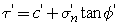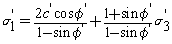Join one of our upcoming courses taking place around the world! Explore now

# Mohr-Coulomb

The Mohr-Coulomb criterion is the most common failure criterion encountered in geotechnical engineering. Many geotechnical analysis methods and programs require use of this strength model. The Mohr-Coulomb criterion describes a linear relationship between normal and shear stresses (or maximum and minimum principal stresses) at failure.

The Mohr-Coulomb criterion implementation in RocData can be used to analyze both direct shear and triaxial test in Define Test Simulations.

The direct shear formulation of the criterion is given by Eqn.1:The Mohr-Coulomb criterion for triaxial data is given by Eqn.2:where c is the cohesive strength, and

is the friction angle.

Equivalent Mohr-Coulomb Parameters

If you are working with one of the non-linear strength criteria in RocData (e.g. Generalized Hoek-Brown, Barton-Bandis or Power Curve), then the equivalent Mohr-Coulomb linear failure envelope is automatically computed over a given stress range. See the Equivalent Mohr-Coulomb Parameters topic for more information.